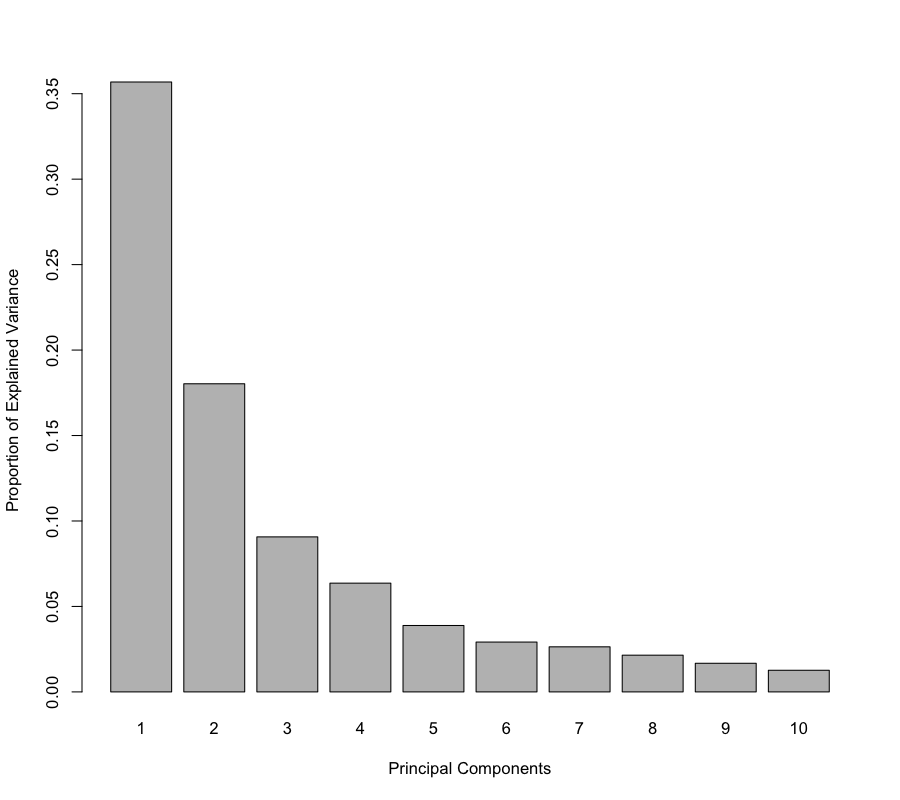# Principal Component Analysis (PCA)

Principal Component Analysis (Jolliffe, 2005) is primarily used to explore one single type of ‘omics data (e.g. transcriptomics, proteomics, metabolomics, etc) and identify the largest sources of variation. PCA is a mathematical procedure that uses orthogonal linear transformation of data from possibly correlated variables into uncorrelated principal components (PCs). The first principal component explains as much of the variability in the data as possible, and each following PC explains as much of the remaining variability as possible. Only the PCs which explain the most variance are retained. This is why choosing the number of dimensions or components (ncomp) is crucial (see the function tune.pca, below).

In mixOmics, PCA is numerically solved in two ways:

1. With singular value decomposition (SVD) of the data matrix,which is the most computationally efficient way and is also adopted by most softwares and the R function prcomp in the stat package.

2. With the Non-linear Iterative Partial Least Squares (NIPALS) in the case of missing values, which uses an iterative power method. See Methods: Missing values.

Both methods are embedded in the mixOmics pca function and will be used accordingly.

Input data should be centered (center = TRUE) and possibly (sometimes preferably) scaled so that all variables have a unit variance. This is especially advised in the case where the variance is not homogeneous across variables (scale = TRUE). By default, the variables are centered and scaled in the function, but the user is free to choose other options.

library(mixOmics)
data(liver.toxicity)
X <- liver.toxicity$gene # Using one data set only  ## Choosing the optimal parameters We can obtain as many dimensions (i.e. number of PCs) as the minimum between the number of samples and variables. However, the goal is to reduce the complexity of the data and therefore summarize the data in fewer underlying dimension. The number of principal Components to retain (also called the number of dimensions) is therefore crucial when performing PCA. The function tune.pca will plot the barplot of the proportion of explained variance for min(n, p)principal components, where n is the number of samples, and p the number of variables. tune.pca(X, ncomp = 10, center = TRUE, scale = FALSE)  ## Eigenvalues for the first 10 principal components, see object$sdev^2:
##        PC1        PC2        PC3        PC4        PC5        PC6
## 17.9714164  9.0792340  4.5677094  3.2043829  1.9567988  1.4686086
##        PC7        PC8        PC9       PC10
##  1.3281206  1.0820554  0.8434155  0.6373565
##
## Proportion of explained variance for the first 10 principal components, see object$explained_variance: ## PC1 PC2 PC3 PC4 PC5 PC6 ## 0.35684128 0.18027769 0.09069665 0.06362638 0.03885429 0.02916076 ## PC7 PC8 PC9 PC10 ## 0.02637122 0.02148534 0.01674690 0.01265538 ## ## Cumulative proportion explained variance for the first 10 principal components, see object$cum.var:
##       PC1       PC2       PC3       PC4       PC5       PC6       PC7
## 0.3568413 0.5371190 0.6278156 0.6914420 0.7302963 0.7594570 0.7858283
##       PC8       PC9      PC10
## 0.8073136 0.8240605 0.8367159
##
##  Other available components:
##  --------------------
##  loading vectors: see object$rotationGiven the barplot output above, we can choose 2 to 3 principal components for the final analysis. # PCA result <- pca(X, ncomp = 3, center = TRUE, scale = FALSE) result  ## Eigenvalues for the first 3 principal components, see object$sdev^2:
##       PC1       PC2       PC3
## 17.971416  9.079234  4.567709
##
## Proportion of explained variance for the first 3 principal components, see object$explained_variance: ## PC1 PC2 PC3 ## 0.35684128 0.18027769 0.09069665 ## ## Cumulative proportion explained variance for the first 3 principal components, see object$cum.var:
##       PC1       PC2       PC3
## 0.3568413 0.5371190 0.6278156
##
##  Other available components:
##  --------------------
##  loading vectors: see object$rotation  # Sparse Principal Component Analysis (sPCA) sPCA (Shen and Huang, 2008) is based on singular value decomposition and is appropriate to deal with large data sets. As implemented in mixOmics, ‘sparsity’ is achieved via LASSO penalizations. sPCA is useful to remove some of the non informative variables in PCA and can be used to investigate whether ‘tighter’ sample clusters can be obtained and which are the variables that highly contribute to each PC. For sPCA, the number of variables to select on each PC must be input by the user ( keepX ). Tuning sPCA keepX based on the amount of explained variance is difficult (the less variables, including noisy variables, the less variance is explained). Since sPCA is an unsupervised and exploratory technique, we prefer to let the user select a keepX suitable to the research question. The following example shows an arbitrary keepX to select the top (10, 5, 15) genes that contribute the most to the variance in the data on the PCs 1, 2 and 3. The function selectVar highlights the variables selected on the comp = 1 PC and outputs their weights in the associated loading vector: spca.result <- spca(X, ncomp = 3, center = TRUE, scale = TRUE, keepX = c(10, 5, 15)) #spca.result selectVar(spca.result, comp = 1)$value

##                 value.var
## A_43_P16829  -0.528762748
## A_42_P680505 -0.428099280
## A_43_P20475  -0.411956357
## A_43_P11409  -0.336141163
## A_43_P21269  -0.331970291
## A_42_P814129 -0.245442052
## A_43_P20891  -0.220272590
## A_43_P20281  -0.175688144
## A_43_P14037  -0.067696801
## A_42_P751969 -0.005174183


# Case study

See Case Study: PCA Multidrug for more examples and plotting options.